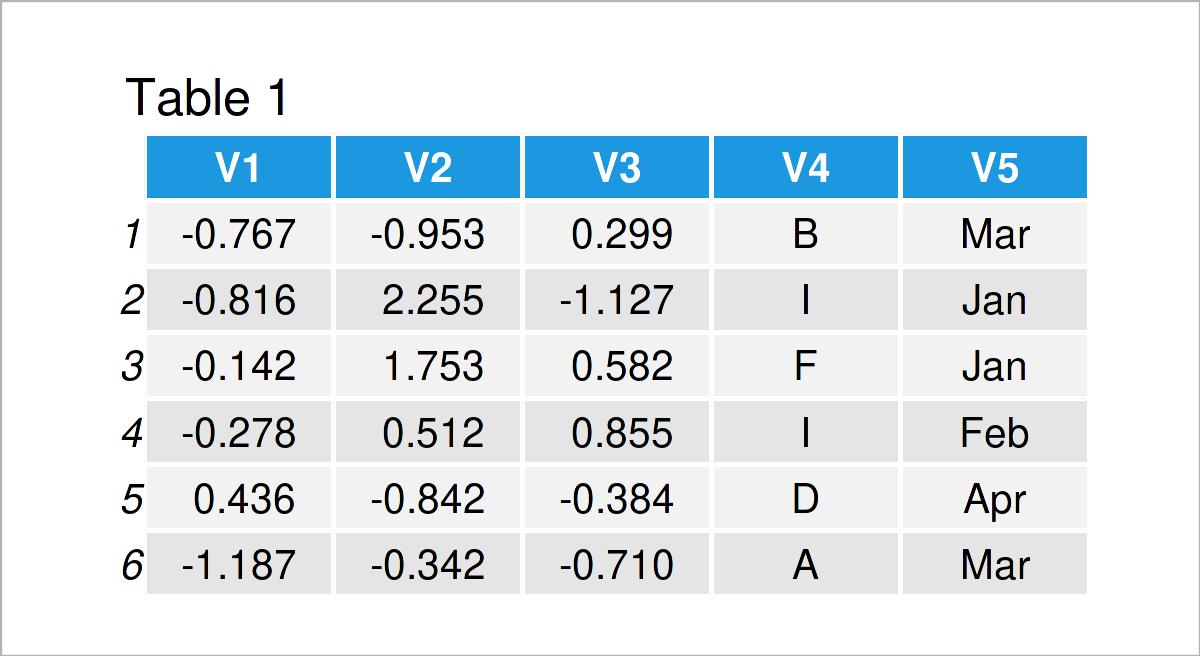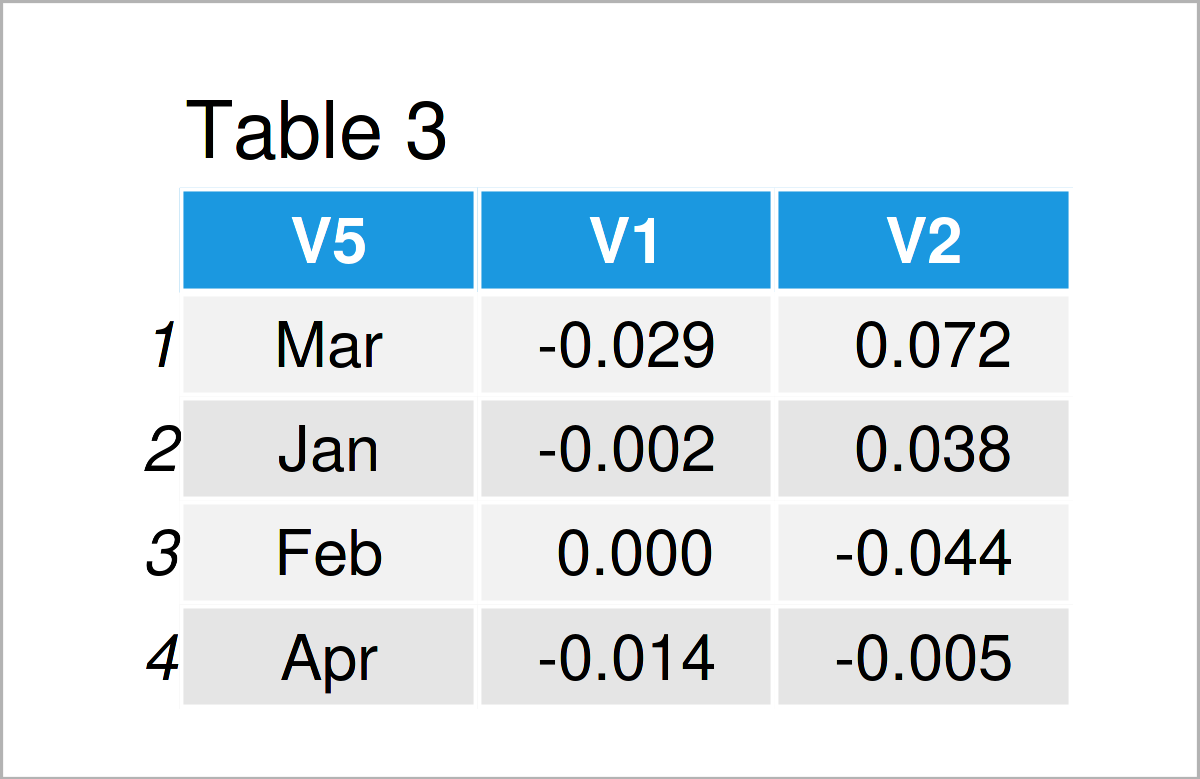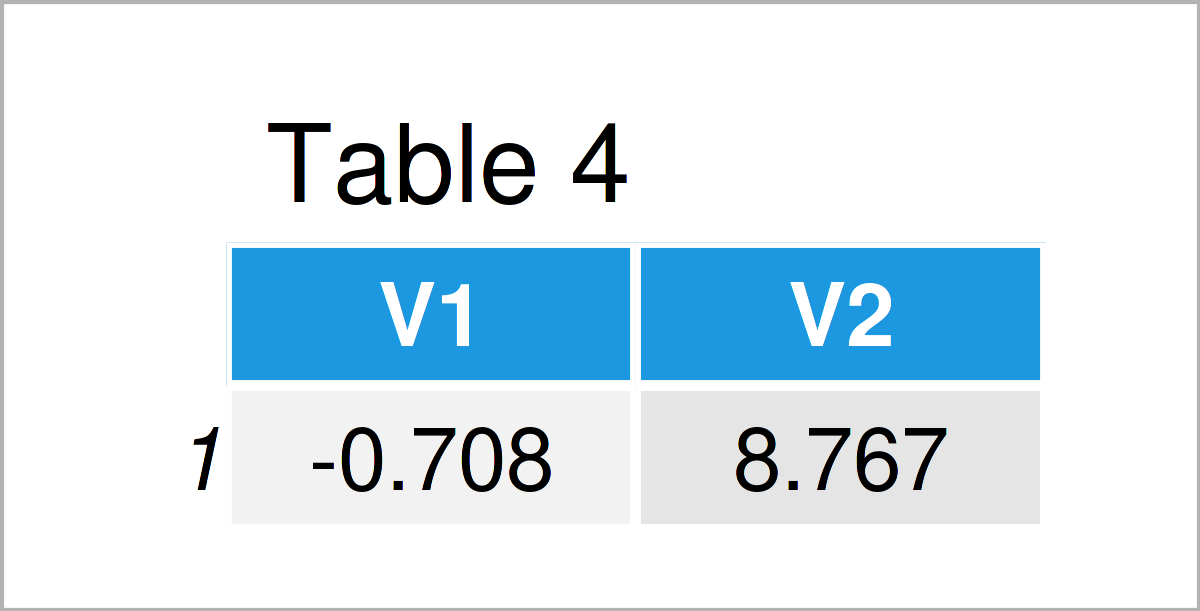# Use lapply Function for data.table in R (4 Examples)

In this post, you’ll learn how to apply a function to multiple columns of a data.table in R programming.

Let’s dive into it…

## Example Data & Software Packages

To be able to use the functions of the data.table package, we first need to install and load data.table:

```install.packages("data.table")              # Install data.table package

For more information on data.table, see our blog post here and the introductory post here.

The following data will be used as a basis for this R tutorial:

```set.seed(9)
data1 <- data.table( V1 = rnorm(500),       # Generate data
V2 = rnorm(500),
V3 = rnorm(500),
V4 = sample(LETTERS[1:10], 50, replace = TRUE),
V5 = sample(month.abb[1:4], 125, replace = TRUE))```
`head(data1)              # Print head of data`As you can see based on Table 1, our example data is a data table containing five columns.

For generating the data, we used some functions. For more information see our post on set.seed() here, rnorm() here, sample() here, LETTERS[] here, month.abb[] here.

## Example 1: Mean Values of Multiple Variables

The following R code illustrates how to apply the mean function to multiple columns of a data.table.

`data1[ , lapply (.SD, mean), .SDcols = c("V1", "V2")] # Calculate mean values`The output of the previous R syntax is shown in Table 2: The mean values of columns V1 and V2. .SD serves as a placeholder of those columns to which a function should be applied to.

## Example 2: Mean Values of Multiple Variables by Groups

In this example, I’ll illustrate how to calculate the mean values of multiple data rows by grouping variable V5, which is a grouping variable with four values.

`data1[ , lapply (.SD, mean), by = .(V5), .SDcols = c("V1", "V2")] # Calculate group means`By executing the previously shown syntax, we have created Table 3. It shows the mean values of V1 and V2 per category of V5.

## Example 3: lapply with Self-Defined Function

In this section, I’ll demonstrate how to use a self-defined function with lapply. You can either define the function before lapply and use it similar to the mean function before. Alternatively, as shown below, you can also define the function within lapply.

`data1[ , lapply (.SD, function (x) { x * 5 }), .SDcols = c("V1", "V2")] # Apply self-defined function`Table 4 shows the output of the previous command.

## Example 4: Define New Variables with lapply

As a last example, we show how to define new data columns V1_new and V2_new within lapply.

```data1 <- data1[ , c("V1_new", "V2_new") := lapply (.SD, function (x) { x^2 }), .SDcols = c("V1", "V2")]  # Define new variablesThe output of the previous R code is shown in Table 5: The two new columns are added as the last columns.

## Video & Further Resources

I have recently published a video on the Statistics Globe YouTube channel, which explains the R codes of this post. You can find the video below.

Furthermore, you may want to have a look at the related articles on my homepage. You can find some articles below.

You have learned in this article how to handle lapply with data.table in R. In case you have any additional questions, tell me about it in the comments section.

This page was created in collaboration with Anna-Lena Wölwer. Have a look at Anna-Lena’s author page to get further information about her academic background and the other articles she has written for Statistics Globe.

Subscribe to the Statistics Globe Newsletter